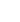15min:
Nuclear spin conversion interaction of the water ion, H2O+, has been studied to derive the spontaneous emission lifetime between the ortho - and para -levels. The H2O+ ion is a radical with 2 B 1 electronic ground state and the off-diagonal electron spin-nuclear spin interaction term, Tab (SaIb + SbIa), connects para and ortho levels, because\boldmath I = \boldmath I1 - \boldmath I2 has nonvanishing matrix elements between I = 0 and 1. The Tab coupling constant, derived by an ab~initio calculation in MRD-CI/Bk level to be 72 MHz, is larger than that of H2O by 4 orders of magnitude, makes the ortho to para conversion of H2O+ faster than that of H2O by 8 orders of magnitude and possibly competitive with other astrophysical processes.
Last year we reported ortho and para coupling channels below 900 cm-1 caused by accidental near degeneracy of rotational levels. For example, hyperfine components of the 42,2(o) and 33,0(p) levels mix each other by 1.2 x 10-3 due to the near degeneracy (E = 0.417 cm-1), but the lower lying 10,1(p) and 11,1(o) levels mix only by 8.9 x 10-5 because of their large separation (E = 16.27 cm-1). In the present study, we solved the radiative rate equations including all the rotational levels below 900 cm-1 to give the o - p conversion lifetime to be 0.451, 3.27, 398 and 910 years for the equilibrium o/p ratio of 3.00, 3.00, 4.52, and 406 when the radiation temperature Tr is 100, 60, 20 and 5 K.
These results qualitatively help to understand the observed high o/p ratio of 4.8 \pm 0.5 (corresponding to the nuclear spin temperature of 21 K) toward Sgr B2, but they are too slow to compete with the reaction by collision unless the number of density of H2 in the region is very low (n1 cm-3) or the radiative temperature is very high (Tr > 50K).